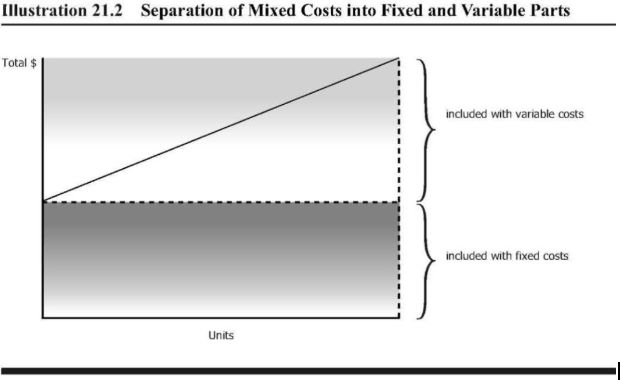## Mixed Costs

### Learning Outcomes

• Define and outline examples of mixed costs in retail and manufacturing business

Mixed costs are those costs that are a combination of fixed and variable costs with elements of both. In a graph form, mixed costs would look like this:Let’s assume that we have a licensing situation, where our base fee is $500 for the first 1,000 widgets, but for each additional widget over 1,000 we sell, we need to pay an additional$1. Looking at the illustration above, the amount included with fixed costs would be $500, since that needs to be paid whether we produce one widget or 5,000 widgets. The variable portion is the$1 per widget.

The equation for mixed costs looks like this:

Y = a + bX

Where:

Y= The total mixed costs

a= The total fixed costs

b= The variable cost per unit of activity (the slope of the line above)

X= The level of activity.

The steeper the slope on the variable line, the higher the variable cost per unit.

What might be a mixed cost in a retail environment? Rent might be a mixed cost. In some leasing situations, there is a base rent, and then a percentage of sales on top of the base. Let’s imagine that you rent a space for a small retail location in your local mall. You are charged a base rent of $500 per month, plus 2% of sales. The fixed portion of this expense is$500, because you pay that amount even if your sales are zero. The variable portion of this expense will be the 2% of sales. For each dollar in sales, you will add .02 to your rent. If you look at the graph above you can see how you apply this graph to our rent example.

## Contribute!

Did you have an idea for improving this content? We’d love your input.